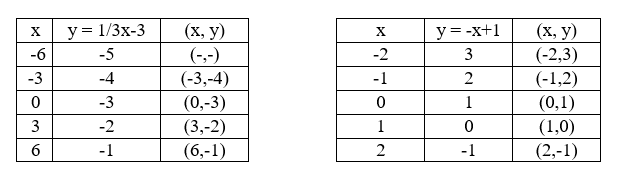Free answers to linear equations with graphs

Recent questions in Linear equations and graphsglamrockqueen7 2021-01-05 Answered

through: (3, 0), parallel to y= -4emancipezN 2021-01-05 Answered

Write an equation of the perpendicular bisector of the segment with the endpoints A(-2,-2) and B(6,0).Harlen Pritchard 2021-01-04 Answered

Given linear equation y = 6 − 7x a. find the y-intercept and slope. b. determine whether the line slopes upward, slopes downward, or is horizontal, without graphing the equation. c. use two points to graph the equation.necessaryh 2021-01-02 Answered

To print his first novel, Shane can use a print-on-demand book printer or he can buy a printer. The book printer charges a setup fee of $150 plus$6 per copy of the book. The printer he would need costs $750, and it would cost$2 per copy to print the book. For how many copies are the costs the same?generals336 2021-01-02 Answered

The parallel lines at the right are cut by a transversal. Find the value of x. a. Angles 1 and 2 are corresponding angles, $$\displaystyle{m}∠{1}={45}°$$, and $$\displaystyle{m}∠{2}={\left({x}+{25}\right)}°$$. b. Angles 3 and 4 are alternate interior angles, $$\displaystyle{m}∠{3}={2}{x}°$$, and $$\displaystyle{m}∠{4}={80}°$$.rocedwrp 2021-01-02 Answered

Solve linear equation and check: $$7x+3=6(x-1)+9$$glamrockqueen7 2020-12-28 Answered

Use what you just learned about negative slopes to solve this problem. A storm moves at a rate of 8 miles per hour. It is 200 miles away from Freeport and headed directly for this town. The equation y = 200 - 8x can be used to represent this function. Identify the slope and y-intercept and explain what they represent.djeljenike 2020-12-28 Answered

Item 11 Graph the linear equation y=3x+1 .emancipezN 2020-12-28 Answered

Determine whether T is a linear transformation. $$\displaystyle{T}:{P}{2}→{P}{2}$$ defined by $$\displaystyle{T}{\left({a}+{b}{x}+{c}{x}^{{{2}}}\right)}={\left({a}+{1}\right)}+{\left({b}+{1}\right)}{x}+{\left({c}+{1}\right)}{x}^{{{2}}}$$Brittney Lord 2020-12-27 Answered

Write the equation of a line perpendicular to $$\displaystyle{y}=\frac{{1}}{{4}}{x}-{5}$$ that passes through the point (-1,2)nicekikah 2020-12-27 Answered

Fill in the blank/s: When solving a system of linear equations by graphing, the system’s solution is determined by locating ___________Mylo O'Moore 2020-12-24 Answered

A rectangular picture frame is twice as long as it is wide. If the area of the frame is $$\displaystyle{288}\in²$$, find its dimensions.defazajx 2020-12-21 Answered

Given linear equation y = -8-4x a. find the y-intercept and slope. b. determine whether the line slopes upward, slopes downward, or is horizontal, without graphing the equation. c. use two points to graph the equation.tricotasu 2020-12-15 Answered

Fill in the bla. a. The graph of a linear equation with two independent variables is a _________. b. The graph of a linear equation with k > 2 independent variables is a _________.Wotzdorfg 2020-12-14 Answered

What would be the linear equation for this function, & the graph? $$\displaystyle{\left({X}_{{1}},{Y}_{{1}}\right)}={\left(-{7},{3}\right)},{\left({X}_{{2}},{Y}_{{2}}\right)}={\left({6},{3}\right)}$$melodykap 2020-12-13 Answered

A system of linear equations is a set of two or more equations taken together. The point where the two graphs intersect is called the solution. $$\displaystyle{\left\lbrace\begin{array}{c} {y}=\frac{{1}}{{3}}{x}-{3}\\{y}=-{x}+{1}\end{array}\right.}$$ a) Complete the table fo each linear functionb) Graph both equations on the coordinate plane belowtinfoQ 2020-12-13 Answered

What would be the linear equation for this function, & the graph?? $$\displaystyle{\left({X}_{{1}},{Y}_{{1}}\right)}={\left({3},{6}\right)},{\left({X}_{{2}},{Y}_{{2}}\right)}={\left({3},-{5}\right)}$$defazajx 2020-12-12 Answered

Find the inverse of the function. y = 3x - 3Chesley 2020-12-09 Answered

Find the x and y intercepts and graph the line. 2x+3y=-9aortiH 2020-12-06 Answered

...